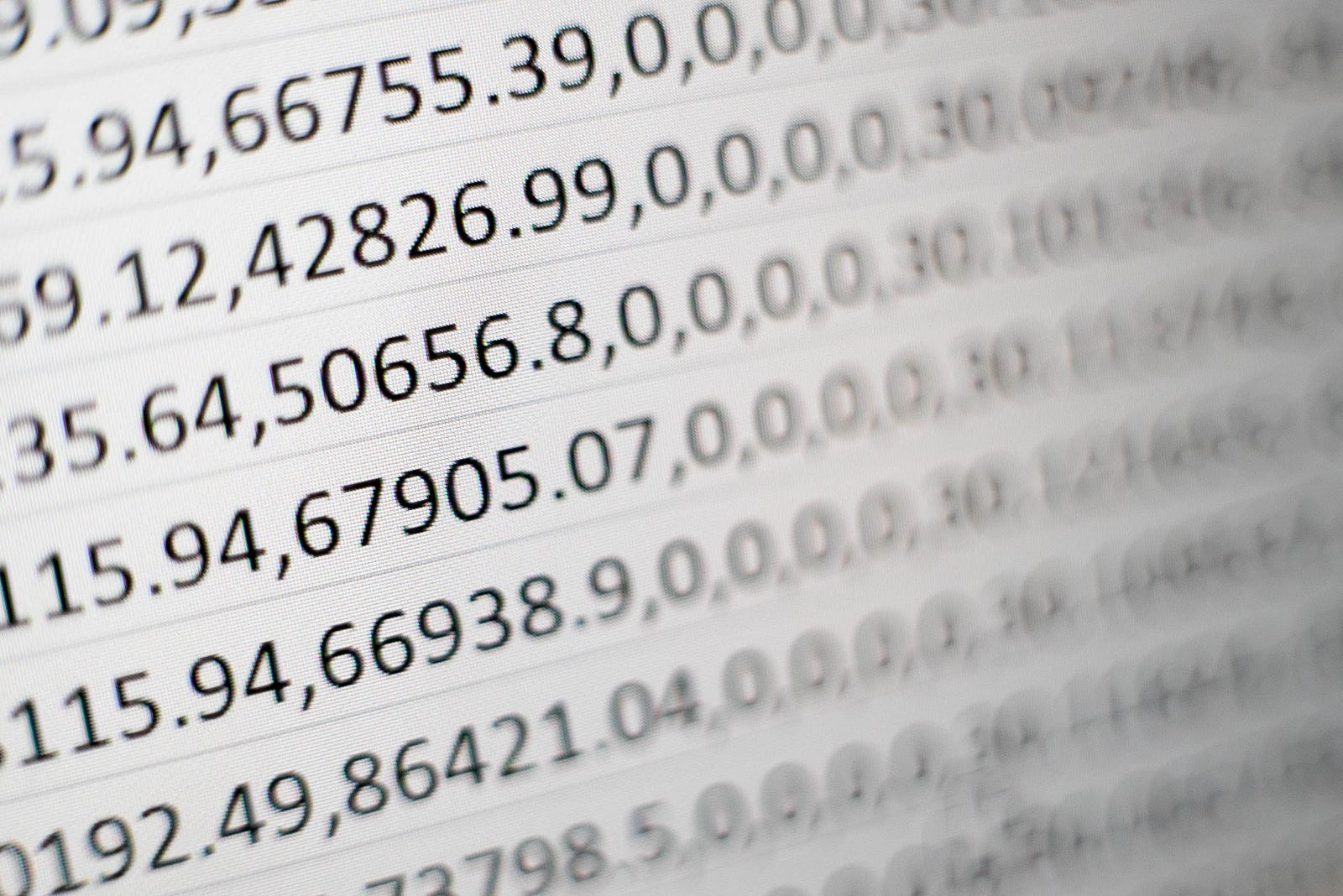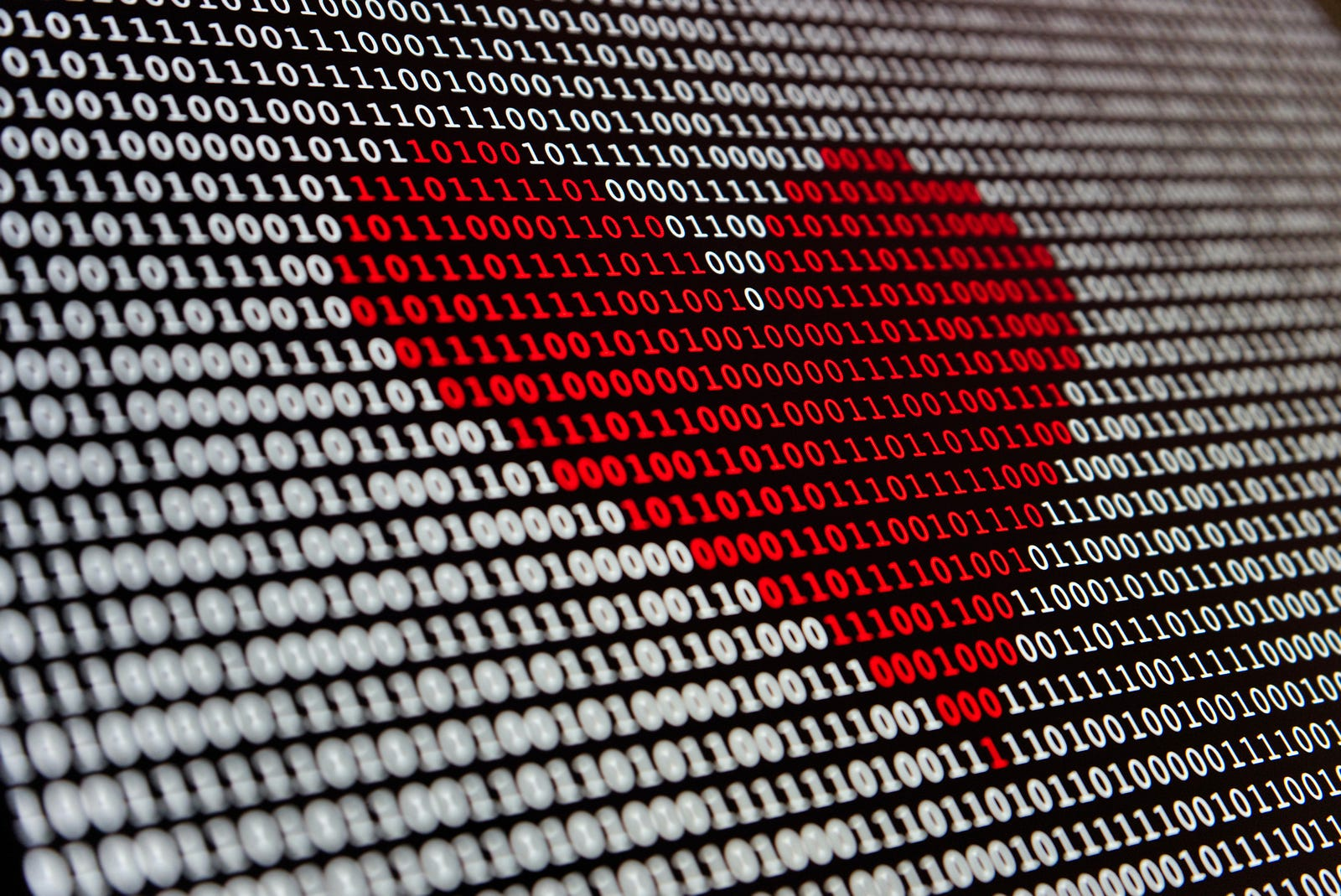# The Mysteries of Floating-Point Numbers

## Exploring the Fascinating World of Computational PrecisionHave you ever wondered why performing simple arithmetic, like multiplying 16.432 by 5.78, seems straightforward when done with pencil and paper, yielding the result 94.97696, but the same calculation in your programming code yields 94.97695999999999?

Welcome to a journey through the intriguing universe of computational precision, where numbers engage in a subtle dance between the realms of floating-point and binary conversion. We'll uncover the secrets of the inherent imprecision in floating-point numbers and understand why seemingly simple operations can yield surprising results. Get ready to discover how the 1985 IEEE 754 standard revolutionized the representation of floating-point numbers and how the conversion from decimals to binaries challenges us to think beyond theory.

### The Evolution of Precision: Focus on Floating-Point

On your quest through computer science, you've likely encountered numbers that, in certain situations, appear somewhat imprecise. Believe it or not, this isn't an isolated peculiarity of a specific programming language but rather an intrinsic characteristic of floating-point numbers in the computational world.Until a certain point in history, floating-point numbers were a bit of a "wild west." Different computer manufacturers and programming languages had unique approaches to representing these numbers. However, in 1985, a game-changer emerged in the world of computing: the IEEE 754 standard. This standard laid the foundation for a uniform representation of floating-point numbers, both at the hardware and software levels. It not only standardized definitions but also introduced different levels of precision, notably single precision and double precision.

### Single Precision and Double Precision: Exploring Beyond Theory

The difference between single precision and double precision is notable. While single precision occupies 32 bits and offers around 7 decimal digits, double precision, with its 64 bits, accommodates approximately 16 decimal digits. The choice between these representations often depends on the programming language and the software's goal. For instance, Python defaults to double precision for its float types, whereas languages like C default to single precision.### Adjusting Precision in Python: An Example

You can adjust floating-point precision in Python by controlling the number of digits after the decimal point. To use double precision, you can do the following:

``````number = 3.14159

# 15 digits after the decimal point

double_precision = "{:.15f}".format(number)

print(double_precision)

For single precision, you can do the following:

number = 3.14159

# 7 digits after the decimal point

single_precision = "{:.7f}".format(number)

print(single_precision)
``````

It's important to note that when adjusting the precision of a floating-point number, truncation occurs rather than rounding. The excess digits are simply discarded.

### Conversion Conflicts: Precision and Rounding Errors

Converting infinite decimal numbers to finite binaries is not as straightforward as it seems. Here, we face crucial decisions: truncate or round to a limited number of binary digits. For example, the binary representation of 1/10 results in a repeating binary fraction (0.0001100110011...), which leads to rounding errors that accumulate during mathematical operations.

### Decimal Library: Taming the Number Dance in Practice

To handle this delicate dance between precision and binary representation, the decimal library comes into play. It offers decimal floating-point arithmetic, allowing you to perform calculations with the necessary precision. Let's see this in action using Python:

``````from decimal import Decimal, getcontext

# Setting the desired precision

getcontext().prec = 20

# Performing a calculation with decimal numbers

result = Decimal('16.432') * Decimal('5.78')

print(result) # Precise result: 94.97696
``````

### Memory Size: The Ocean in a Bottle Metaphor

Imagine trying to fit an ocean into a small bottle. It's nearly impossible to contain the vastness of the ocean within a confined space. Similarly, attempting to represent infinite numbers within a predefined floating-point size is like trying to fit the ocean into a bottle. This metaphor visualizes the complex task of balancing precision with available resources.It's worth noting that using the decimal library, while offering precision, may demand more computational resources and impact performance. Just as in the dance of numbers, finding the balance between precision and performance is crucial for obtaining satisfactory results.

### Conclusion: Navigating the Number Dance

Our exploration of the imprecision of floating-point numbers and conversion concludes. You are now the captain of this numerical journey, leading the numbers with curiosity and practice. Remember that, as numbers dance between decimal and binary, the decimal library is your ally. Armed with this knowledge, you navigate confidently through the sea of computational mathematics, understanding the intricate dance that unfolds before your eyes.